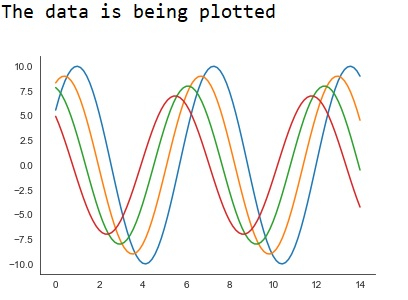# How can seaborn library be used to display data without the background axis spines in Python?

Machine learning deals with creating models from data, and generalizing on never before seen data. The data provided to a machine learning model as input should be such that it should be understood by the system properly, so that it can interpret the data and produce results.

Visualizing data is an important step since it helps understand what is going on in the data without actually looking at the numbers and performing complicated computations.

This interface helps in customizing and controlling the kind of data and how it behaves when certain filters are applied to it. The ‘despine’ function can be used to remove the background axis spines while displaying the data visually on the console. Let us see an example to remove the background axis spines −

## Example

import numpy as np
from matplotlib import pyplot as plt
def sin_plot(flip=1):
x = np.linspace(0, 14, 99)
for i in range(1, 5):
plt.plot(x, np.sin(x + i * .59) * (11 - i) * flip)
import seaborn as sb
sb.set_style("white")
print("The data is being plotted ")
sin_plot()
sb.despine()
plt.show()

## Output## Explanation

• The required packages are imported.
• The input data is generated using the user defined function named ‘sine_plot’.
• The ‘despine’ function is used to remove the background axis spines from the plot.
• This data is specified to be plotted using seaborn library
• This visual data is displayed on the console.

Updated on: 11-Dec-2020

81 Views